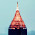星期四, 四月 14, 2011

层次分析法在R语言中的实现

1、建立层次结构模型。在深入分析实际问题的基础上，将有关的各个因素按照不同属性自上而下地分解成若干层次，同一层的诸因素从属于上一层的因素或对上层因素有影响，同时又支配下一层的因素或受到下层因素的作用。最上层为目标层，通常只有1个因素，最下层通常为方案或对象层，中间可以有一个或几个层次，通常为准则或指标层。当准则过多时(譬如多于9个)应进一步分解出子准则层。

2、构造成对比较阵。从层次结构模型的第2层开始，对于从属于(或影响)上一层每个因素的同一层诸因素，用成对比较法和1—9比较尺度构造成对比较阵，直到最下层。

3、计算权向量并做一致性检验。对于每一个成对比较阵计算最大特征根及对应特征向量，利用一致性指标、随机一致性指标和一致性比率做一致性检验。若检验通过，特征向量(归一化后)即为权向量：若不通过，需重新构造成对比较阵。

4、计算组合权向量并做组合一致性检验。计算最下层对目标的组合权向量，并根据公式做组合一致性检验，若检验通过，则可按照组合权向量表示的结果进行决策，否则需要重新考虑模型或重新构造那些一致性比率较大的成对比较阵。

function (B)
{
A = matrix(B, nrow = sqrt(length(B)), ncol = sqrt(length(B)),
byrow = TRUE)
n = ncol(A)
mul_collect = c(1:n)
for (i in 1:n) mul_collect[i] = prod(A[i, ])
weight = mul_collect^(1/n)
weight_one = weight/sum(weight)
round(weight_one, 4)
}

function (B)
{
RI = c(0, 0, 0.58, 0.9, 1.12, 1.24, 1.32, 1.41, 1.45, 1.49,
1.51)
Wi = weight(B)
n = length(Wi)
if (n > 2) {
W = matrix(Wi, ncol = 1)
A = matrix(B, nrow = sqrt(length(B)), ncol = sqrt(length(B)),
byrow = TRUE)
AW = A %*% W
aw = as.vector(AW)
la_max = sum(aw/Wi)/n
CI = (la_max - n)/(n - 1)
CR = CI/RI[n]
cat("\n CI=", round(CI, 4), "\n")
cat("\n CR=", round(CR, 4), "\n")
cat("\n la_max=", round(la_max, 4), "\n\n")
if (CR <= 0.1) {
cat(" 通过一致性检验！\n")
cat("\n Wi: ", round(Wi, 4), "\n")
}
else {
cat(" 请调整判断矩阵！\n")
Wi = null
break
}
}
else if (n <= 2) {
return(Wi)
}
}

1 条评论:

1.我用MBA智库百科里面的数据（例2）来测试
http://wiki.mbalib.com/wiki/层次分析法
权值与MBA智库百科的结果不同